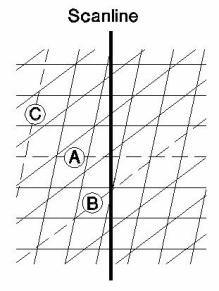# Terzaghi Weighting

The Terzaghi Weighting option can be applied to Contour and Rosette plots to account for the sampling bias introduced by orientation data collection along Traverses.

To apply or remove the Terzaghi weighting:

• Select the Terzaghi weighting checkbox in the Sidebar plot options, OR
• You can also select the Terzaghi Weighting option in the View menu. This will display a dialog which also allows you to specify the Minimum Bias Angle (see below for details).

When orientation measurements are made, a bias is introduced in favour of those features which are perpendicular to the direction of surveying. To illustrate this concept, three joints of identical spacing along a scanline are shown below.Measurements along the scanline record many more joints in Set A than in Set C, which will bias the density contour plot heavily in favour of Set A. To compensate for this bias, a geometrical weighting factor is calculated and applied to each feature measured. This weighting, W, can be applied to contour and rosette plots in Dips, and is also used in the weighted mean vector calculations. The bias correction should only be used for planar features, and will not account properly for measurement bias in linear features such as acicular crystal fabric.

The geometric weighting factor, W, is calculated as follows:a = minimum angle between plane and Traverse D’ = apparent spacing along Traverse D = D’ sin a = D’ (1/W) = true spacing of discontinuity set R’ = 1/D = 1/D’ sin a = D’ cosec a = true density of joint population W = (1) cosec a = weighting applied to individual pole before density calculation

Since the weighting function tends to infinity as alpha ( a) approaches zero, a maximum limit for this weighting must be set to prevent unreasonable results. This maximum limit corresponds to a minimum angle, which can be between 1° and 89.9°. However, the recommended range is limited to 5° to 25°, and the default is set to 15°. The user can change this limit with the Minimum Bias Angle option (see below).

The effect of applying the Terzaghi Weighting to some data distributions can be quite severe. If you use weighted data plots for design or interpretation, be sure you understand the weighting procedure.

The results of applying the weighting procedure to a sample data file are shown in the following figure. In this case, there is a very important subnormal group of joints, which is masked by abundant structural data collected on horizontal scanlines. In this case, the heavy bias introduced by the horizontal data can be removed by weighting the data.Illustration of Terzaghi Weighting – unweighted (left) and weighted (right) contours of sample data.

Note that the Terzaghi Weighting option is only enabled if you have defined Traverses in your Dips file (i.e. if at least one Traverse has been defined in the Traverse Information dialog). If no traverses exist, then the Terzaghi Weighting option is not applicable and will not be available.

The Terzaghi Weighting only affects the apparent concentration of poles shown on contour (and rosette) plots. The Terzaghi Weighting does not change the location of the poles, which represent your raw data.

You can obtain the actual Terzaghi Weighting factor which has been applied to the poles by using the Save Processed File option. This allows you to save a processed version of your Dips file. If you select the Terzaghi Weighting checkbox in the dialog that appears, the processed file will contain an extra column with the actual Terzaghi Weighting value which has been applied to each measurement.

## Minimum Bias Angle

When you select the Terzaghi Weighting option from the View menu, you will see a dialog which allows you to apply the Terzaghi Weighting, and also to specify a Minimum Bias Angle. The Minimum Bias Angle is actually a limit used by the Terzaghi correction, to prevent the weighting factor from becoming excessively large. It is set to a default value of 15 degrees. The maximum possible weighting factor corresponds to the Minimum Bias Angle. Any planes which intersect a Traverse orientation at an angle less than the Minimum Bias Angle, will be limited to the maximum weighting factor.